## Similarity-Based Remaining Useful Life Estimation

This example shows how to build a complete Remaining Useful Life (RUL) estimation workflow including the steps for preprocessing, selecting trendable features, constructing a health indicator by sensor fusion, training similarity RUL estimators, and validating prognostics performance. The example uses the training data from the PHM2008 challenge dataset from https://ti.arc.nasa.gov/tech/dash/groups/pcoe/prognostic-data-repository/ .

### Data Preparation

Since the dataset is small it is feasible to load the whole degradation data into memory. Download and unzip the data set from https://ti.arc.nasa.gov/tech/dash/groups/pcoe/prognostic-data-repository/ to the current directory. Use the `helperLoadData` helper function to load and convert the training text file to a cell array of timetables. The training data contains 218 run-to-failure simulations. This group of measurements is called an "ensemble".

```degradationData = helperLoadData('train.txt'); degradationData(1:5)```
```ans = 5×1 cell array {223×26 table} {164×26 table} {150×26 table} {159×26 table} {357×26 table} ```

Each ensemble member is a table with 26 columns. The columns contain data for the machine ID, time stamp, 3 operating conditions and 21 sensor measurements.

`head(degradationData{1})`
```ans=8×26 table id time op_setting_1 op_setting_2 op_setting_3 sensor_1 sensor_2 sensor_3 sensor_4 sensor_5 sensor_6 sensor_7 sensor_8 sensor_9 sensor_10 sensor_11 sensor_12 sensor_13 sensor_14 sensor_15 sensor_16 sensor_17 sensor_18 sensor_19 sensor_20 sensor_21 __ ____ ____________ ____________ ____________ ________ ________ ________ ________ ________ ________ ________ ________ ________ _________ _________ _________ _________ _________ _________ _________ _________ _________ _________ _________ _________ 1 1 10.005 0.2501 20 489.05 604.13 1499.5 1310 10.52 15.49 394.88 2318.9 8770.2 1.26 45.4 372.15 2388.1 8120.8 8.6216 0.03 368 2319 100 28.58 17.174 1 2 0.0015 0.0003 100 518.67 642.13 1584.5 1404 14.62 21.61 553.67 2388 9045.8 1.3 47.29 521.81 2388.2 8132.9 8.3907 0.03 391 2388 100 38.99 23.362 1 3 34.999 0.8401 60 449.44 555.42 1368.2 1122.5 5.48 8 194.93 2222.9 8343.9 1.02 41.92 183.26 2387.9 8063.8 9.3557 0.02 334 2223 100 14.83 8.8555 1 4 20.003 0.7005 0 491.19 607.03 1488.4 1249.2 9.35 13.65 334.82 2323.8 8721.5 1.08 44.26 314.84 2388.1 8052.3 9.2231 0.02 364 2324 100 24.42 14.783 1 5 42.004 0.8405 40 445 549.52 1354.5 1124.3 3.91 5.71 138.24 2211.8 8314.6 1.02 41.79 130.44 2387.9 8083.7 9.2986 0.02 330 2212 100 10.99 6.4025 1 6 20.003 0.7017 0 491.19 607.37 1480.5 1258.9 9.35 13.65 334.51 2323.9 8711.4 1.08 44.4 315.36 2388.1 8053.2 9.2276 0.02 364 2324 100 24.44 14.702 1 7 42 0.84 40 445 549.57 1354.4 1131.4 3.91 5.71 139.11 2211.8 8316.9 1.02 42.09 130.16 2387.9 8082 9.3753 0.02 331 2212 100 10.53 6.4254 1 8 0.0011 0 100 518.67 642.08 1589.5 1407.6 14.62 21.61 553.48 2388.1 9050.4 1.3 47.5 521.74 2388 8133.3 8.4339 0.03 391 2388 100 38.98 23.234 ```

Split the degradation data into a training data set and a validation data set for later performance evaluation.

```rng('default') % To make sure the results are repeatable numEnsemble = length(degradationData); numFold = 5; cv = cvpartition(numEnsemble, 'KFold', numFold); trainData = degradationData(training(cv, 1)); validationData = degradationData(test(cv, 1));```

Specify groups of variables of interest.

```varNames = string(degradationData{1}.Properties.VariableNames); timeVariable = varNames{2}; conditionVariables = varNames(3:5); dataVariables = varNames(6:26);```

Visualize a sample of the ensemble data.

```nsample = 10; figure helperPlotEnsemble(trainData, timeVariable, ... [conditionVariables(1:2) dataVariables(1:2)], nsample)```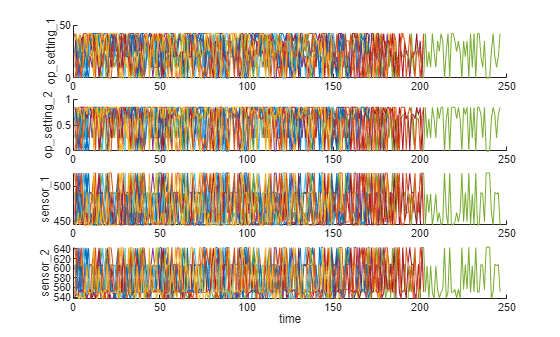### Working Regime Clustering

As shown in the previous section, there is no clear trend showing the degradation process in each run-to-failure measurement. In this and the next section, the operating conditions will be used to extract clearer degradation trends from sensor signals.

Notice that each ensemble member contains 3 operating conditions: "op_setting_1", "op_setting_2", and "op_setting_3". First, let's extract the table from each cell and concatenate them into a single table.

```trainDataUnwrap = vertcat(trainData{:}); opConditionUnwrap = trainDataUnwrap(:, cellstr(conditionVariables));```

Visualize all operating points on a 3D scatter plot. It clearly shows 6 regimes and the points in each regime are in very close proximity.

```figure helperPlotClusters(opConditionUnwrap)```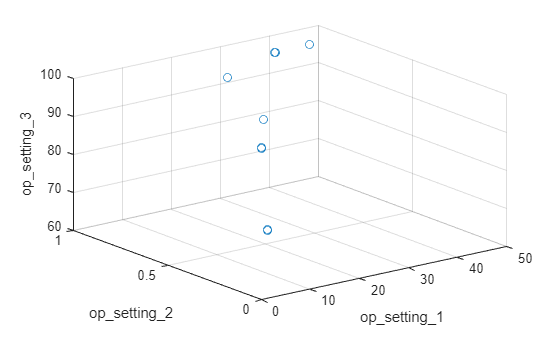Let's use clustering techniques to locate the 6 clusters automatically. Here, the K-means algorithm is used. K-means is one of the most popular clustering algorithms, but it can result in local optima. It is a good practice to repeat the K-means clustering algorithm several times with different initial conditions and pick the results with the lowest cost. In this case, the algorithm runs 5 times and the results are identical.

```opts = statset('Display', 'final'); [clusterIndex, centers] = kmeans(table2array(opConditionUnwrap), 6, ... 'Distance', 'sqeuclidean', 'Replicates', 5, 'Options', opts);```
```Replicate 1, 1 iterations, total sum of distances = 0.279547. Replicate 2, 1 iterations, total sum of distances = 0.279547. Replicate 3, 1 iterations, total sum of distances = 0.279547. Replicate 4, 1 iterations, total sum of distances = 0.279547. Replicate 5, 1 iterations, total sum of distances = 0.279547. Best total sum of distances = 0.279547 ```

Visualize the clustering results and the identified cluster centroids.

```figure helperPlotClusters(opConditionUnwrap, clusterIndex, centers)```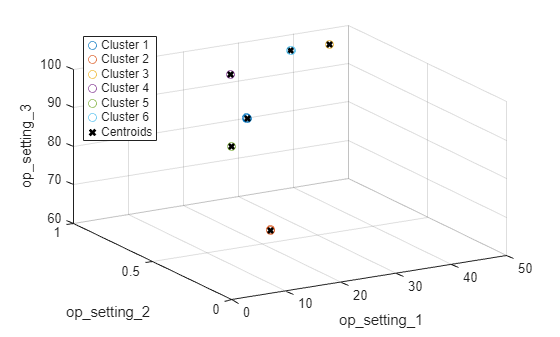As the plot illustrates, the clustering algorithm successfully finds the 6 working regimes.

### Working Regime Normalization

Let's perform a normalization on measurements grouped by different working regimes. First, compute the mean and standard deviation of each sensor measurement grouped by the working regimes identified in the last section.

```centerstats = struct('Mean', table(), 'SD', table()); for v = dataVariables centerstats.Mean.(char(v)) = splitapply(@mean, trainDataUnwrap.(char(v)), clusterIndex); centerstats.SD.(char(v)) = splitapply(@std, trainDataUnwrap.(char(v)), clusterIndex); end centerstats.Mean```
```ans=6×21 table sensor_1 sensor_2 sensor_3 sensor_4 sensor_5 sensor_6 sensor_7 sensor_8 sensor_9 sensor_10 sensor_11 sensor_12 sensor_13 sensor_14 sensor_15 sensor_16 sensor_17 sensor_18 sensor_19 sensor_20 sensor_21 ________ ________ ________ ________ ________ ________ ________ ________ ________ _________ _________ _________ _________ _________ _________ _________ _________ _________ _________ _________ _________ 489.05 604.92 1502.1 1311.4 10.52 15.493 394.32 2319 8784.1 1.26 45.496 371.44 2388.2 8133.9 8.6653 0.03 369.74 2319 100 28.525 17.115 518.67 642.71 1590.7 1409.4 14.62 21.61 553.3 2388.1 9062.3 1.3 47.557 521.36 2388.1 8140.9 8.4442 0.03 393.27 2388 100 38.809 23.285 462.54 536.87 1262.8 1050.6 7.05 9.0275 175.4 1915.4 8014.9 0.93989 36.808 164.56 2028.3 7878 10.916 0.02 307.39 1915 84.93 14.262 8.5552 445 549.72 1354.7 1128.2 3.91 5.7158 138.62 2212 8327 1.0202 42.163 130.53 2388 8088.4 9.3775 0.02 331.12 2212 100 10.584 6.3515 491.19 607.59 1486 1253.6 9.35 13.657 334.46 2324 8729.1 1.0777 44.466 314.85 2388.2 8065 9.2347 0.022299 365.45 2324 100 24.447 14.67 449.44 555.82 1366.9 1131.9 5.48 8.0003 194.43 2223 8355.2 1.0203 41.995 183.01 2388.1 8071.1 9.3344 0.02 334.29 2223 100 14.827 8.8966 ```
`centerstats.SD`
```ans=6×21 table sensor_1 sensor_2 sensor_3 sensor_4 sensor_5 sensor_6 sensor_7 sensor_8 sensor_9 sensor_10 sensor_11 sensor_12 sensor_13 sensor_14 sensor_15 sensor_16 sensor_17 sensor_18 sensor_19 sensor_20 sensor_21 __________ ________ ________ ________ __________ _________ ________ ________ ________ __________ _________ _________ _________ _________ _________ __________ _________ _________ __________ _________ _________ 1.4553e-11 0.47617 5.8555 8.3464 1.1618e-12 0.0047272 0.65536 0.094487 18.057 1.51e-13 0.25089 0.52838 0.096183 16.012 0.037598 9.9929e-16 1.4723 0 0 0.14522 0.086753 4.059e-11 0.48566 5.9258 8.8223 3.7307e-14 0.0011406 0.86236 0.068654 20.061 1.2103e-13 0.25937 0.71239 0.069276 17.212 0.036504 9.9929e-16 1.5046 0 0 0.17565 0.10515 2.7685e-11 0.35468 5.2678 6.9664 2.043e-14 0.0043301 0.45074 0.2743 14.741 0.0010469 0.21539 0.33985 0.28932 13.624 0.044142 8.6744e-16 1.3083 0 5.9833e-12 0.11212 0.06722 0 0.44169 5.6853 7.5741 1.9763e-13 0.0049401 0.44198 0.30713 18.389 0.0014951 0.23584 0.3432 0.33144 16.917 0.037135 2.231e-15 1.4174 0 0 0.10778 0.063991 4.4456e-11 0.46992 5.7664 7.8679 8.9892e-13 0.0046633 0.59984 0.13032 17.983 0.0042388 0.2362 0.49055 0.13285 15.792 0.038156 0.0042084 1.4428 0 0 0.13352 0.079731 4.3489e-11 0.44341 5.7224 7.4842 3.7485e-13 0.0017642 0.4734 0.28889 17.608 0.0017978 0.23242 0.38243 0.31 15.91 0.038624 8.5009e-16 1.3974 0 0 0.11311 0.069348 ```

The statistics in each regime can be used to normalize the training data. For each ensemble member, extract the operating points of each row, compute its distance to each cluster centers and find the nearest cluster center. Then, for each sensor measurement, subtract the mean and divide it by the standard deviation of that cluster. If the standard deviation is close to 0, set the normalized sensor measurement to 0 because a nearly constant sensor measurement is not useful for remaining useful life estimation. Refer to the last section, "Helper Functions", for more details on regimeNormalization function.

```trainDataNormalized = cellfun(@(data) regimeNormalization(data, centers, centerstats), ... trainData, 'UniformOutput', false);```

Visualize the data normalized by working regime. Degradation trends for some sensor measurements are now revealed after normalization.

```figure helperPlotEnsemble(trainDataNormalized, timeVariable, dataVariables(1:4), nsample)```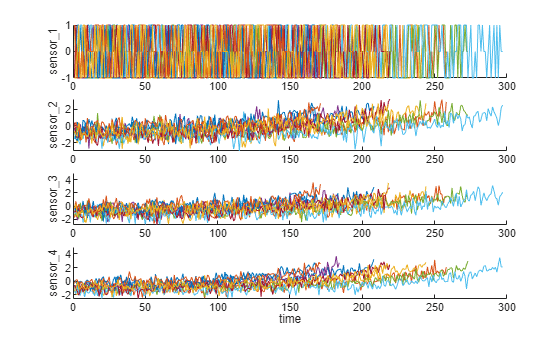### Trendability Analysis

Now select the most trendable sensor measurements to construct a health indicator for prediction. For each sensor measurement, a linear degradation model is estimated and the slopes of the signals are ranked.

```numSensors = length(dataVariables); signalSlope = zeros(numSensors, 1); warn = warning('off'); for ct = 1:numSensors tmp = cellfun(@(tbl) tbl(:, cellstr(dataVariables(ct))), trainDataNormalized, 'UniformOutput', false); mdl = linearDegradationModel(); % create model fit(mdl, tmp); % train mode signalSlope(ct) = mdl.Theta; end warning(warn);```

Sort the signal slopes and select 8 sensors with the largest slopes.

```[~, idx] = sort(abs(signalSlope), 'descend'); sensorTrended = sort(idx(1:8))```
```sensorTrended = 8×1 2 3 4 7 11 12 15 17 ```

Visualize the selected trendable sensor measurements.

```figure helperPlotEnsemble(trainDataNormalized, timeVariable, dataVariables(sensorTrended(3:6)), nsample)```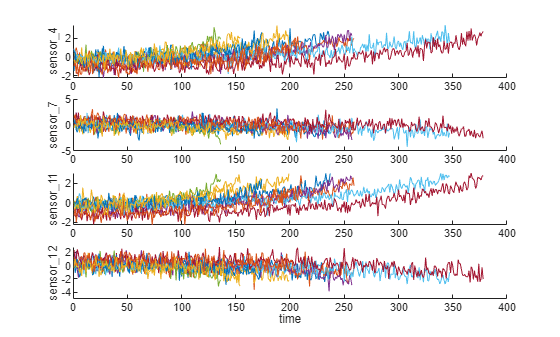Notice that some of the most trendable signals show positive trends, while others show negative trends.

### Construct Health Indicator

This section focuses on fusing the sensor measurements into a single health indicator, with which a similarity-based model is trained.

All the run-to-failure data is assumed to start with a healthy condition. The health condition at the beginning is assigned a value of 1 and the health condition at failure is assigned a value of 0. The health condition is assumed to be linearly degrading from 1 to 0 over time. This linear degradation is used to help fuse the sensor values. More sophisticated sensor fusion techniques are described in the literature [2-5].

```for j=1:numel(trainDataNormalized) data = trainDataNormalized{j}; rul = max(data.time)-data.time; data.health_condition = rul / max(rul); trainDataNormalized{j} = data; end```

Visualize the health condition.

```figure helperPlotEnsemble(trainDataNormalized, timeVariable, "health_condition", nsample)```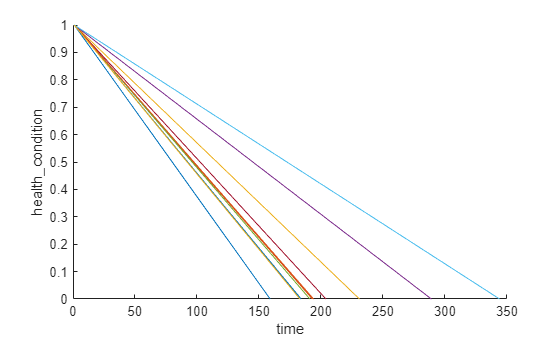The health condition of all ensemble members change from 1 to 0 with varying degrading speeds.

Now fit a linear regression model of Health Condition with the most trended sensor measurements as regressors:

Health Condition ~ 1 + Sensor2 + Sensor3 + Sensor4 + Sensor7 + Sensor11 + Sensor12 + Sensor15 + Sensor17

```trainDataNormalizedUnwrap = vertcat(trainDataNormalized{:}); sensorToFuse = dataVariables(sensorTrended); X = trainDataNormalizedUnwrap{:, cellstr(sensorToFuse)}; y = trainDataNormalizedUnwrap.health_condition; regModel = fitlm(X,y); bias = regModel.Coefficients.Estimate(1)```
```bias = 0.5000 ```
`weights = regModel.Coefficients.Estimate(2:end)`
```weights = 8×1 -0.0308 -0.0308 -0.0536 0.0033 -0.0639 0.0051 -0.0408 -0.0382 ```

Construct a single health indicator by multiplying the sensor measurements with their associated weights .

```trainDataFused = cellfun(@(data) degradationSensorFusion(data, sensorToFuse, weights), trainDataNormalized, ... 'UniformOutput', false);```

Visualize the fused health indicator for training data.

```figure helperPlotEnsemble(trainDataFused, [], 1, nsample) xlabel('Time') ylabel('Health Indicator') title('Training Data')```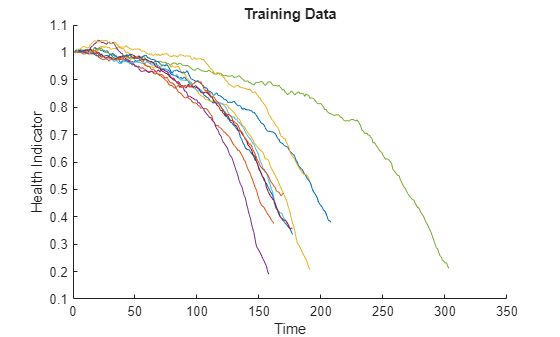The data from multiple sensors are fused into a single health indicator. The health indicator is smoothed by a moving average filter. See helper function "degradationSensorFusion" in the last section for more details.

### Apply same operation to validation data

Repeat the regime normalization and sensor fusion process with the validation data set.

```validationDataNormalized = cellfun(@(data) regimeNormalization(data, centers, centerstats), ... validationData, 'UniformOutput', false); validationDataFused = cellfun(@(data) degradationSensorFusion(data, sensorToFuse, weights), ... validationDataNormalized, 'UniformOutput', false);```

Visualize the health indicator for validation data.

```figure helperPlotEnsemble(validationDataFused, [], 1, nsample) xlabel('Time') ylabel('Health Indicator') title('Validation Data')```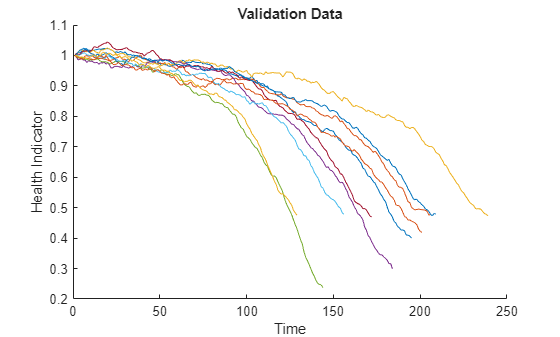### Build Similarity RUL Model

Now build a residual-based similarity RUL model using the training data. In this setting, the model tries to fit each fused data with a 2nd order polynomial.

The distance between data $\mathit{i}$ and data $\mathit{j}$ is computed by the 1-norm of the residual

`$d\left(i,j\right)=||{y}_{j}-{\underset{}{\overset{ˆ}{y}}}_{j,i}|{|}_{1}$`

where ${\mathit{y}}_{\mathit{j}}$ is the health indicator of machine $\mathit{j}$, ${\underset{}{\overset{ˆ}{y}}}_{j,i}$ is the estimated health indicator of machine $\mathit{j}$ using the 2nd order polynomial model identified in machine $\mathit{i}$.

The similarity score is computed by the following formula

`$score\left(i,j\right)=exp\left(-d\left(i,j{\right)}^{2}\right)$`

Given one ensemble member in the validation data set, the model will find the nearest 50 ensemble members in the training data set, fit a probability distribution based on the 50 ensemble members, and use the median of the distribution as an estimate of RUL.

```mdl = residualSimilarityModel(... 'Method', 'poly2',... 'Distance', 'absolute',... 'NumNearestNeighbors', 50,... 'Standardize', 1); fit(mdl, trainDataFused);```

### Performance Evaluation

To evaluate the similarity RUL model, use 50%, 70% and 90% of a sample validation data to predict its RUL.

```breakpoint = [0.5, 0.7, 0.9]; validationDataTmp = validationDataFused{3}; % use one validation data for illustration```

Use the validation data before the first breakpoint, which is 50% of the lifetime.

```bpidx = 1; validationDataTmp50 = validationDataTmp(1:ceil(end*breakpoint(bpidx)),:); trueRUL = length(validationDataTmp) - length(validationDataTmp50); [estRUL, ciRUL, pdfRUL] = predictRUL(mdl, validationDataTmp50);```

Visualize the validation data truncated at 50% and its nearest neighbors.

```figure compare(mdl, validationDataTmp50);```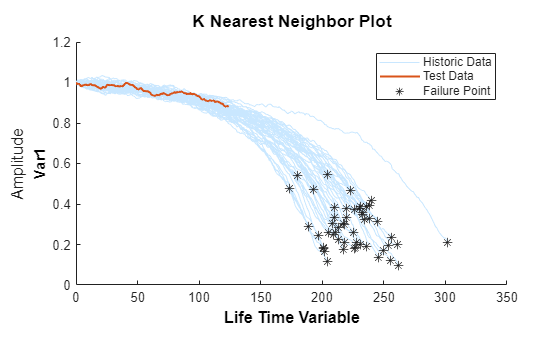Visualize the estimated RUL compared to the true RUL and the probability distribution of the estimated RUL.

```figure helperPlotRULDistribution(trueRUL, estRUL, pdfRUL, ciRUL)```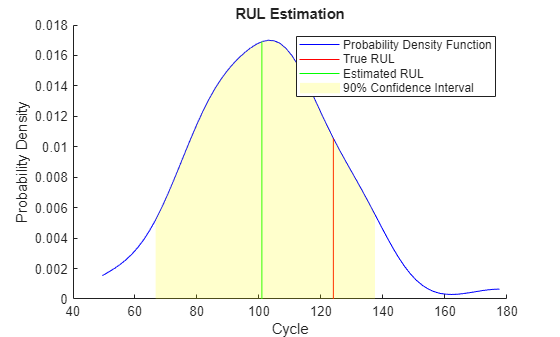There is a relatively large error between the estimated RUL and the true RUL when the machine is in an intermediate health stage. In this example, the most similar 10 curves are close at the beginning, but bifurcate when they approach the failure state, resulting in roughly two modes in the RUL distribution.

Use the validation data before the second breakpoint, which is 70% of the lifetime.

```bpidx = 2; validationDataTmp70 = validationDataTmp(1:ceil(end*breakpoint(bpidx)), :); trueRUL = length(validationDataTmp) - length(validationDataTmp70); [estRUL,ciRUL,pdfRUL] = predictRUL(mdl, validationDataTmp70); figure compare(mdl, validationDataTmp70);```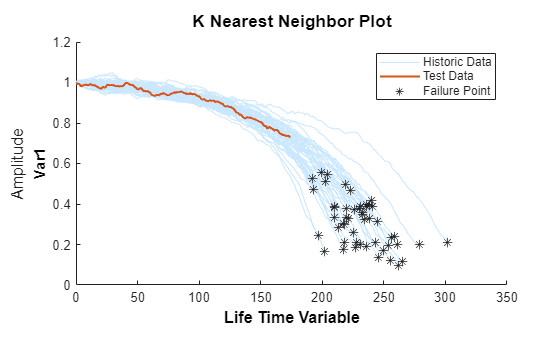```figure helperPlotRULDistribution(trueRUL, estRUL, pdfRUL, ciRUL)```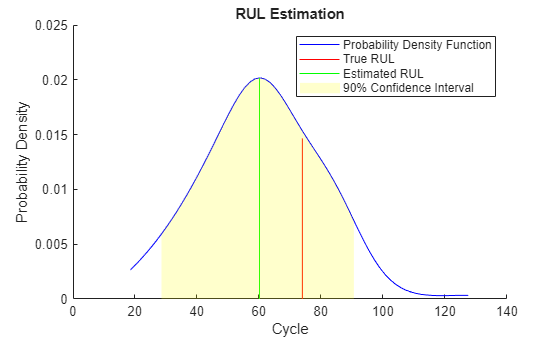When more data is observed, the RUL estimation is enhanced.

Use the validation data before the third breakpoint, which is 90% of the lifetime.

```bpidx = 3; validationDataTmp90 = validationDataTmp(1:ceil(end*breakpoint(bpidx)), :); trueRUL = length(validationDataTmp) - length(validationDataTmp90); [estRUL,ciRUL,pdfRUL] = predictRUL(mdl, validationDataTmp90); figure compare(mdl, validationDataTmp90);```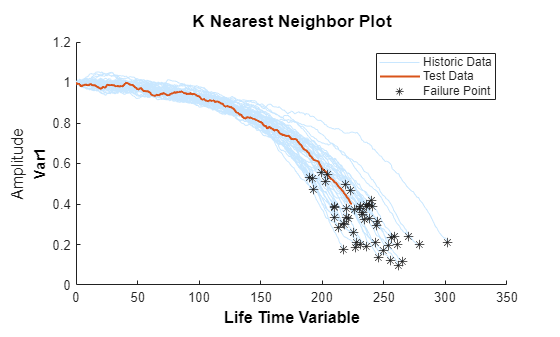```figure helperPlotRULDistribution(trueRUL, estRUL, pdfRUL, ciRUL)```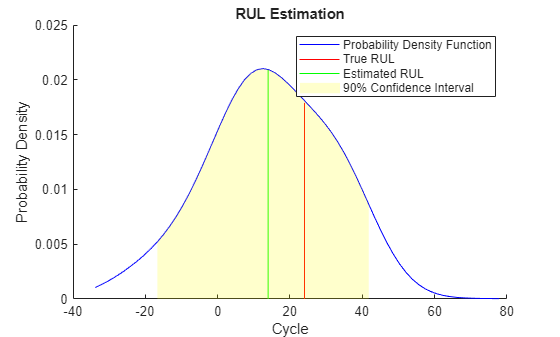When the machine is close to failure, the RUL estimation is even more enhanced in this example.

Now repeat the same evaluation procedure for the whole validation data set and compute the error between estimated RUL and true RUL for each breakpoint.

```numValidation = length(validationDataFused); numBreakpoint = length(breakpoint); error = zeros(numValidation, numBreakpoint); for dataIdx = 1:numValidation tmpData = validationDataFused{dataIdx}; for bpidx = 1:numBreakpoint tmpDataTest = tmpData(1:ceil(end*breakpoint(bpidx)), :); trueRUL = length(tmpData) - length(tmpDataTest); [estRUL, ~, ~] = predictRUL(mdl, tmpDataTest); error(dataIdx, bpidx) = estRUL - trueRUL; end end```

Visualize the histogram of the error for each breakpoint together with its probability distribution.

```[pdf50, x50] = ksdensity(error(:, 1)); [pdf70, x70] = ksdensity(error(:, 2)); [pdf90, x90] = ksdensity(error(:, 3)); figure ax(1) = subplot(3,1,1); hold on histogram(error(:, 1), 'BinWidth', 5, 'Normalization', 'pdf') plot(x50, pdf50) hold off xlabel('Prediction Error') title('RUL Prediction Error using first 50% of each validation ensemble member') ax(2) = subplot(3,1,2); hold on histogram(error(:, 2), 'BinWidth', 5, 'Normalization', 'pdf') plot(x70, pdf70) hold off xlabel('Prediction Error') title('RUL Prediction Error using first 70% of each validation ensemble member') ax(3) = subplot(3,1,3); hold on histogram(error(:, 3), 'BinWidth', 5, 'Normalization', 'pdf') plot(x90, pdf90) hold off xlabel('Prediction Error') title('RUL Prediction Error using first 90% of each validation ensemble member') linkaxes(ax)```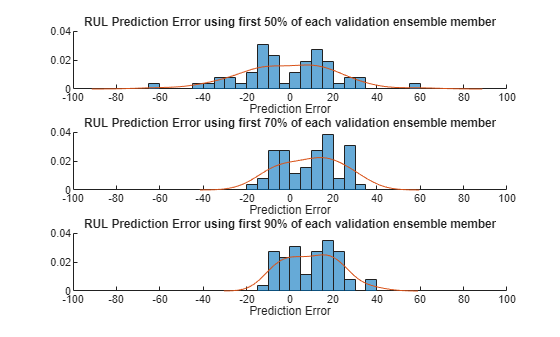Plot the prediction error in a box plot to visualize the median, 25-75 quantile and outliers.

```figure boxplot(error, 'Labels', {'50%', '70%', '90%'}) ylabel('Prediction Error') title('Prediction error using different percentages of each validation ensemble member')```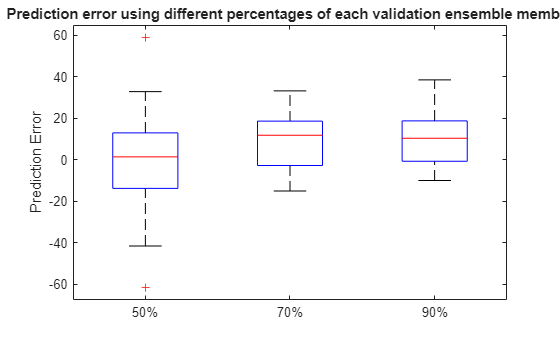Compute and visualize the mean and standard deviation of the prediction error.

`errorMean = mean(error)`
```errorMean = 1×3 -5.8944 3.1359 3.3555 ```
`errorMedian = median(error)`
```errorMedian = 1×3 -4.8538 5.3763 3.6580 ```
`errorSD = std(error)`
```errorSD = 1×3 26.4916 20.0720 18.0313 ```
```figure errorbar([50 70 90], errorMean, errorSD, '-o', 'MarkerEdgeColor','r') xlim([40, 100]) xlabel('Percentage of validation data used for RUL prediction') ylabel('Prediction Error') legend('Mean Prediction Error with 1 Standard Deviation Eror bar', 'Location', 'south')```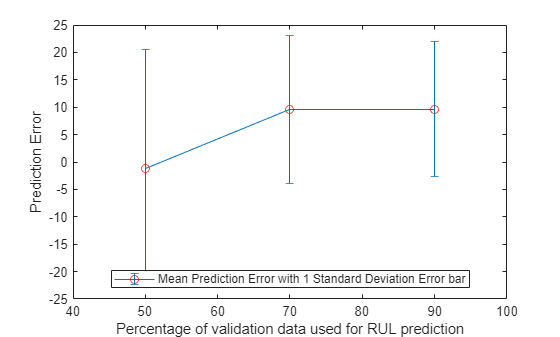It is shown that the error becomes more concentrated around 0 (less outliers) as more data is observed.

### References

 A. Saxena and K. Goebel (2008). "PHM08 Challenge Data Set", NASA Ames Prognostics Data Repository (http://ti.arc.nasa.gov/project/prognostic-data-repository), NASA Ames Research Center, Moffett Field, CA

 Roemer, Michael J., Gregory J. Kacprzynski, and Michael H. Schoeller. "Improved diagnostic and prognostic assessments using health management information fusion." AUTOTESTCON Proceedings, 2001. IEEE Systems Readiness Technology Conference. IEEE, 2001.

 Goebel, Kai, and Piero Bonissone. "Prognostic information fusion for constant load systems." Information Fusion, 2005 8th International Conference on. Vol. 2. IEEE, 2005.

 Wang, Peng, and David W. Coit. "Reliability prediction based on degradation modeling for systems with multiple degradation measures." Reliability and Maintainability, 2004 Annual Symposium-RAMS. IEEE, 2004.

 Jardine, Andrew KS, Daming Lin, and Dragan Banjevic. "A review on machinery diagnostics and prognostics implementing condition-based maintenance." Mechanical systems and signal processing 20.7 (2006): 1483-1510.

### Helper Functions

```function data = regimeNormalization(data, centers, centerstats) % Perform normalization for each observation (row) of the data % according to the cluster the observation belongs to. conditionIdx = 3:5; dataIdx = 6:26; % Perform row-wise operation data{:, dataIdx} = table2array(... rowfun(@(row) localNormalize(row, conditionIdx, dataIdx, centers, centerstats), ... data, 'SeparateInputs', false)); end function rowNormalized = localNormalize(row, conditionIdx, dataIdx, centers, centerstats) % Normalization operation for each row. % Get the operating points and sensor measurements ops = row(1, conditionIdx); sensor = row(1, dataIdx); % Find which cluster center is the closest dist = sum((centers - ops).^2, 2); [~, idx] = min(dist); % Normalize the sensor measurements by the mean and standard deviation of the cluster. % Reassign NaN and Inf to 0. rowNormalized = (sensor - centerstats.Mean{idx, :}) ./ centerstats.SD{idx, :}; rowNormalized(isnan(rowNormalized) | isinf(rowNormalized)) = 0; end function dataFused = degradationSensorFusion(data, sensorToFuse, weights) % Combine measurements from different sensors according % to the weights, smooth the fused data and offset the data % so that all the data start from 1 % Fuse the data according to weights dataToFuse = data{:, cellstr(sensorToFuse)}; dataFusedRaw = dataToFuse*weights; % Smooth the fused data with moving mean stepBackward = 10; stepForward = 10; dataFused = movmean(dataFusedRaw, [stepBackward stepForward]); % Offset the data to 1 dataFused = dataFused + 1 - dataFused(1); end```